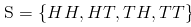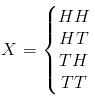Courses

# Probability Distribution (Part - 1) Mathematics Notes | EduRev

## Mathematics : Probability Distribution (Part - 1) Mathematics Notes | EduRev

The document Probability Distribution (Part - 1) Mathematics Notes | EduRev is a part of the Mathematics Course Additional Topics for IIT JAM Mathematics.
All you need of Mathematics at this link: Mathematics

Introduction to Probability Distributions - Random Variables
A random variable is defined as a function that associates a real number (the probability value) to an outcome of an experiment.
In other words, a random variable is a generalization of the outcomes or events in a given sample space. This is possible since the random variable by definition can change so we can use the same variable to refer to different situations. Random variables make working with probabilities much neater and easier.
A random variable in probability is most commonly denoted by capital X, and the small letter x is then used to ascribe a value to the random variable.
For examples, given that you flip a coin twice, the sample space for the possible outcomes is given by the following:There are four possible outcomes as listed in the sample space above; where H stands for heads and T stands for tails.
The random variable X can be given by the following:To find the probability of one of those out comes we denote that question as:
P(X = x)
which means that the probability that the random variable is equal to some real number x.
In the above example, we can say:
Let X be a random variable defined as the number of heads obtained when two coins are tossed. Find the probability the you obtain two heads.
So now we've been told what X is and that x = 2, so we write the above information as:
P(X = 2)
Since we already have the sample space, we know that there is only one outcomes with two heads, so we find the probability as:
P(X = 2) = 1/4
we can also simply write the above as:
P(X) = 1/4
From this example, you should be able to see that the random variable X refers to any of the elements in a given sample space.
There are two types of random variables: discrete variables and continuous random variables.

Discrete Random Variables
The word discrete means separate and individual. Thus discrete random variables are those that take on integer values only. They never include fractions or decimals.
A quick example is the sample space of any number of coin flips, the outcomes will always be integer values, and you'll never have half heads or quarter tails. Such a random variable is referred to as discrete. Discrete random variables give rise to discrete probability distributions.

Continuous Random Variable
Continuous is the opposite of discrete. Continuous random variables are those that take on any value including fractions and decimals. Continuous random variables give rise to continuous probability distributions.

Offer running on EduRev: Apply code STAYHOME200 to get INR 200 off on our premium plan EduRev Infinity!

,

,

,

,

,

,

,

,

,

,

,

,

,

,

,

,

,

,

,

,

,

;